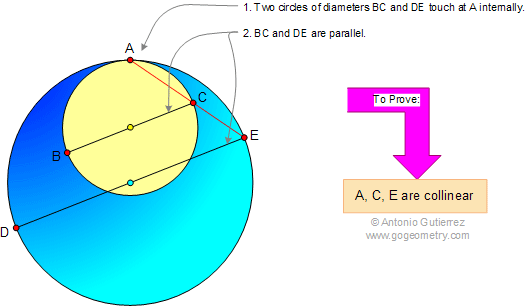Problem 640. Archimedes' Book of Lemmas: Proposition 1. Level: High School, SAT Prep, College geometry. 1. Internally Tangent Circles at A If two circles touch at A (internally), and if BC and DE are parallel diameters, then ACE is a straight line.2. Externally Tangent Circles at A If two circles touch at A (externally), and if BC and DE are parallel diameters, then ACE is a straight line.See also: Etching and Typography of Lemma 1 Recent Additions